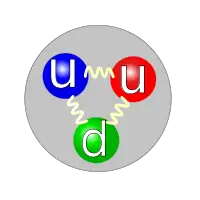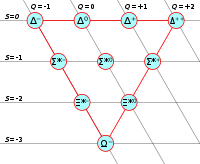# BaryonA proton is an example of a baryon. It is composed of 2 up quarks (u) and 1 down quark (d).

The term baryon usually refers to a subatomic particle composed of three quarks. A more technical (and broader) definition is that it is a subatomic particle with a baryon number of 1. Baryons are a subset of hadrons, (which are particles made of quarks), and they participate in the strong interaction. They are also a subset of fermions. Well-known examples of baryons are protons and neutrons, which make up atomic nuclei, but many unstable baryons have been found as well.

Some "exotic" baryons, known as pentaquarks, are thought to be composed of four quarks and one antiquark, but their existence is not generally accepted. Each baryon has a corresponding antiparticle, called an anti-baryon, in which quarks are replaced by their corresponding antiquarks.

## Etymology

The term baryon is derived from the Greek word βαρύς (barys), meaning "heavy," because at the time of their naming it was believed that baryons were characterized by having greater mass than other particles.

## Basic properties

Each baryon has an odd half-integer spin (such as 12 or 32), where "spin" refers to the angular momentum quantum number. Baryons are therefore classified as fermions. They experience the strong nuclear force and are described by Fermi-Dirac statistics, which apply to all particles obeying the Pauli exclusion principle. This stands in contrast to bosons, which do not obey the exclusion principle.

Baryons, along with mesons, are hadrons, meaning they are particles composed of quarks. Each quark has a baryon number of B = 13, and each antiquark has a baryon number of B = −13.

The term baryon number is defined as:

$B={\frac {N_{q}-N_{\overline {q}}}{3}}$where

$N_{q}\$is the number of quarks, and
$N_{\overline {q}}$is the number of antiquarks.

The term "baryon" is usually used for triquarks, that is, baryons made of three quarks. Thus, each baryon has a baryon number of 1 (B = 13 + 13 + 13 = 1).

Some have suggested the existence of other, "exotic" baryons, such as pentaquarks—baryons made of four quarks and one antiquark (B = 13 + 13 + 13 + 13 − 13 = 1)—but their existence is not generally accepted. Theoretically, heptaquarks (5 quarks, 2 antiquarks), nonaquarks (6 quarks, 3 antiquarks), and so forth could also exist.

Besides being associated with a spin number and a baryon number, each baryon has a quantum number known as strangeness. This quantity is equal to -1 times the number of strange quarks present in the baryon.

## ClassificationCombinations of three u, d or s-quarks with a total spin of 3/2 form the so-called baryon decuplet.

Baryons are classified into groups according to their isospin values and quark content. There are six groups of triquarks:

• Nucleon (N)
• Delta (Δ)
• Lambda (Λ)
• Sigma (Σ)
• Xi (Ξ)
• Omega (Ω)

The rules for classification are defined by the Particle Data Group. The rules cover all the particles that can be made from three of each of the six quarks (up, down, strange, charm, bottom, top), although baryons made of top quarks are not expected to exist because of the top quark's short lifetime. (The rules do not cover pentaquarks.) According to these rules, the u, d, and s quarks are considered light, and the c, b, and t quarks are considered heavy.

Based on the rules, the following classification system has been set up:

• Baryons with three u and/or d quarks are grouped as N (isospin 12) or Δ (isospin 32).
• Baryons with two u and/or d quarks are grouped as Λ (isospin 0) or Σ (isospin 1). If the third quark is heavy, its identity is given by a subscript.
• Baryons with one u or d quark are placed in the group Ξ (isospin 12). One or two subscripts are used if one or both of the remaining quarks are heavy.
• Baryons with no u or d quarks are placed in the group Ω (isospin 0), and subscripts indicate any heavy quark content.
• Some baryons decay strongly, in which case their masses are shown as part of their names. For example, Sigmas (Σ) and Omegas (Ω) do not decay strongly, but Deltas (Δ(1232)), and charmed Xis (Ξ+c(2645)) do.

Given that quarks carry charge, knowledge of the charge of a particle indirectly gives the quark content. For example, the rules say that the Σb contains a bottom and some combination of two up and/or down quarks. A Σ0b must be one up quark (Q=23), one down quark (Q=−13), and one bottom quark (Q=−13) to have the correct charge (Q=0).

The number of baryons within one group (excluding resonances) is given by the number of isospin projections possible (2 × isospin + 1). For example, there are four Δ's, corresponding to the four isospin projections of the isospin value I = 32: Δ++ (Iz = 32), Δ+(Iz = 12), Δ0(Iz = −12), and Δ(Iz = −32). Another example would be the three Σb's, corresponding to the three isospin projections of the isospin value I = 1: Σ+b (Iz = 1), Σ0b(Iz = 0), and Σb(Iz = −1).

### Charmed baryons

Baryons that are composed of at least one charm quark are known as charmed baryons.

## Baryonic matter

Baryonic matter is matter composed mostly of baryons (by mass). It includes atoms of all types, and thus includes nearly all types of matter that we may encounter or experience in everyday life, including the matter that constitutes human bodies. Non-baryonic matter, as implied by the name, is any sort of matter that is not primarily composed of baryons. It may include such ordinary matter as neutrinos or free electrons, but it may also include exotic species of non-baryonic dark matter, such as supersymmetric particles, axions, or black holes.

The distinction between baryonic and non-baryonic matter is important in cosmology, because Big Bang nucleosynthesis models set tight constraints on the amount of baryonic matter present in the early universe.

The very existence of baryons is also a significant issue in cosmology because current theory assumes that the Big Bang produced a state with equal amounts of baryons and anti-baryons. The process by which baryons came to outnumber their antiparticles is called baryogenesis. (This is distinct from a process by which leptons account for the predominance of matter over antimatter, known as leptogenesis.)

## Baryogenesis

Experiments are consistent with the number of quarks in the universe being a constant and, more specifically, the number of baryons being a constant; in technical language, the total baryon number appears to be conserved. Within the prevailing Standard Model of particle physics, the number of baryons may change in multiples of three due to the action of sphalerons, although this is rare and has not been observed experimentally. Some grand unified theories of particle physics also predict that a single proton can decay, changing the baryon number by one; however, this has not yet been observed experimentally. The excess of baryons over antibaryons in the present universe is thought to be due to non-conservation of baryon number in the very early universe, though this is not well understood.